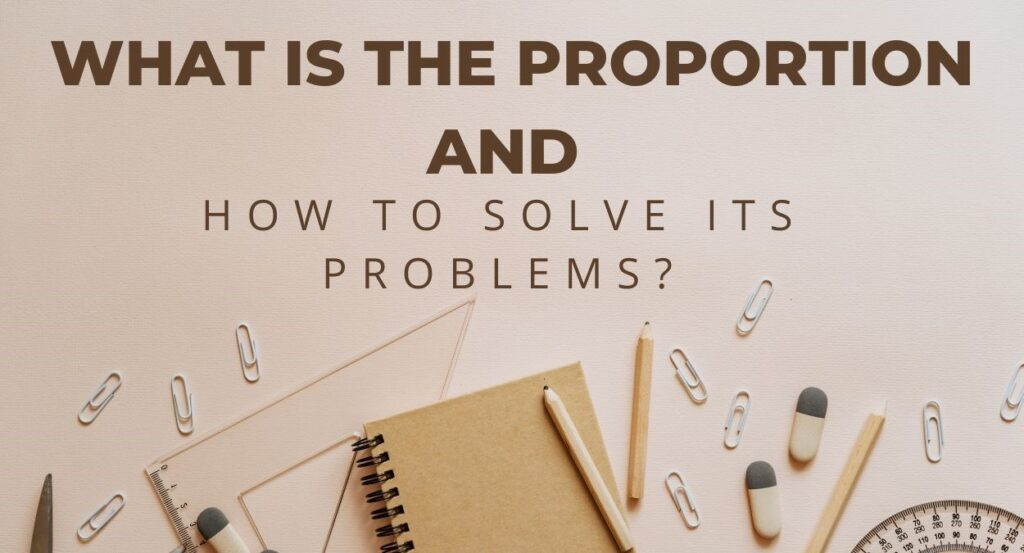## What is the Proportion and How to Solve its Problems?

Proportion is based on fractions. The fraction is written as a/b and the ratio is a: b, whereas the proportion is defined as the equality between two ratios. Proportions are a very important concept in mathematics and other social sciences. In real life, the concept of proportions is used in business when we deal with …